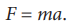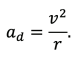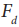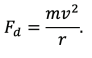# Centripetal force

Newton's second law states that if a force of magnitude ? acts on a body of mass ?, it causes acceleration ? according to the relation:f an object with mass ? moves evenly along a path with radius of curvature ?, the direction of movement of the object changes, although the magnitude of the speed does not change ("only" its direction). This fact is expressed by the so called centripetal acceleration ??, for which it is possible to derive the relation:Centripetal forceis then equal to:filter_none